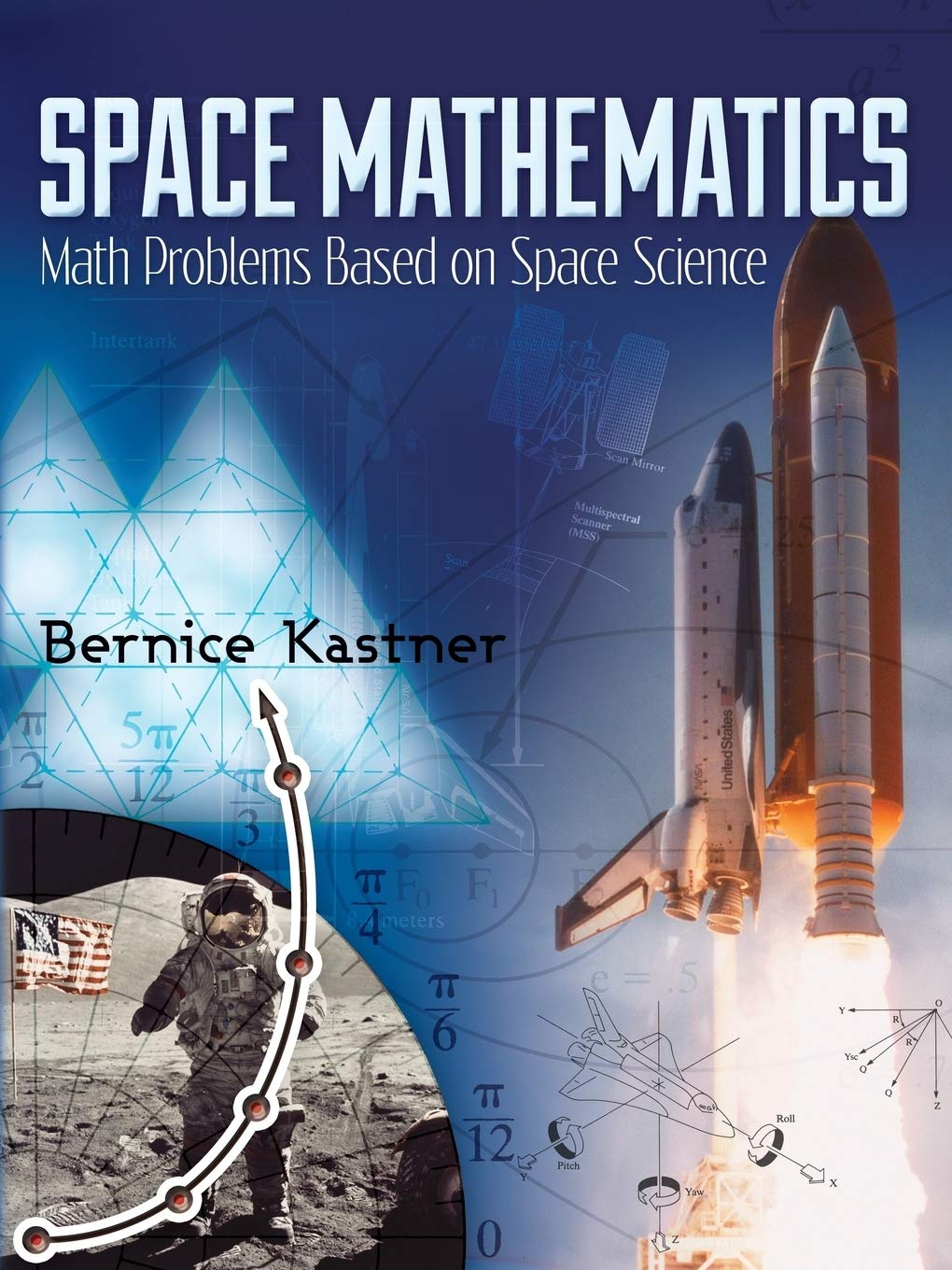# Space Mathematics: Math Problems Based on Space Science (Dover Books on Aeronautical Engineering)

\$9.99

Price: \$9.99
(as of Feb 17,2021 16:54:49 UTC – Details)

Created by NASA for high school students interested in space science, this collection of worked problems covers a broad range of subjects, including mathematical aspects of NASA missions, computation and measurement, algebra, geometry, probability and statistics, exponential and logarithmic functions, trigonometry, matrix algebra, conic sections, and calculus. In addition to enhancing mathematical knowledge and skills, these problems promote an appreciation of aerospace technology and offer valuable insights into the practical uses of secondary school mathematics by professional scientists and engineers.
Geared toward high school students and teachers, this volume also serves as a fine review for undergraduate science and engineering majors. Numerous figures illuminate the text, and an appendix explores the advanced topic of gravitational forces and the conic section trajectories.Space Mathematics: Math Problems Based on Space Science (Dover Books on Aeronautical Engineering)

\$9.99